# 6. Let f be a 20-periodic function, such that f(x) = x2 on (-1, ). The...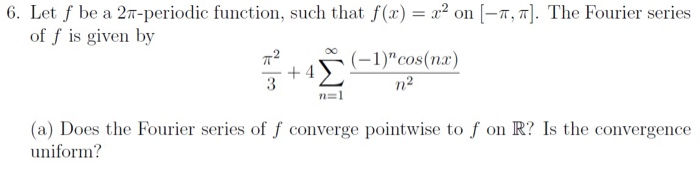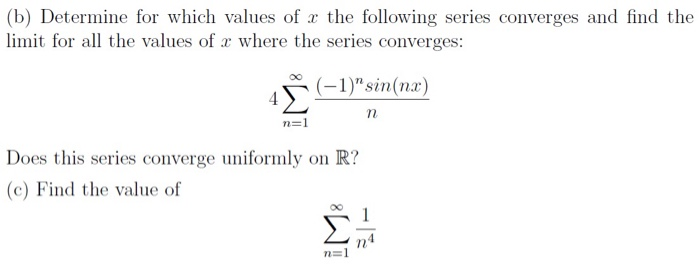6. Let f be a 20-periodic function, such that f(x) = x2 on (-1, ). The Fourier series of f is given by 72 (-1)"cos(nx) n2 +4 3 n=1 (a) Does the Fourier series of f converge pointwise to f on R? Is the convergence uniform?
(b) Determine for which values of x the following series converges and find the limit for all the values of x where the series converges: (-1)"sin(nx) n n=1 Does this series converge uniformly on R? (c) Find the value of n4 n=1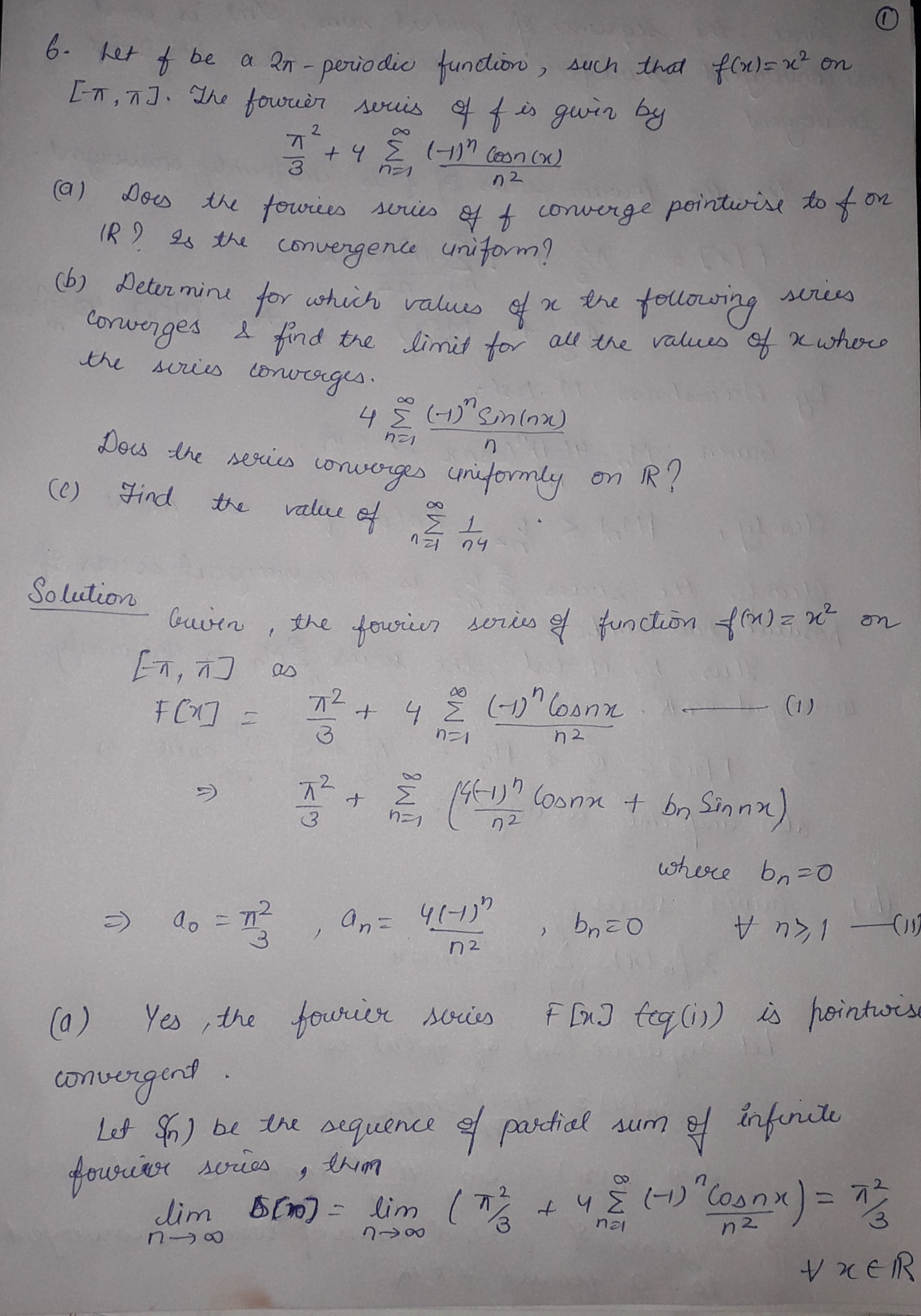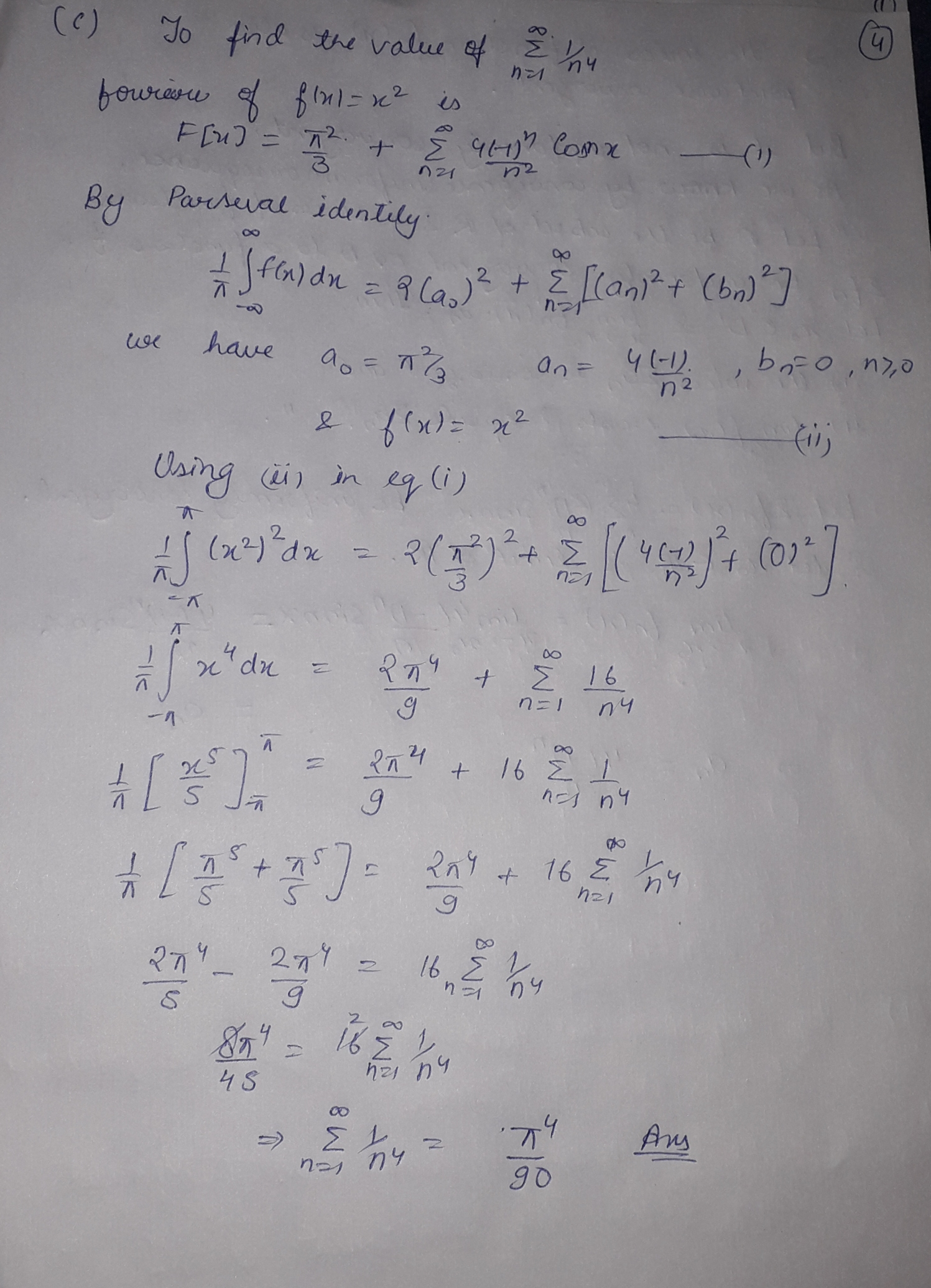p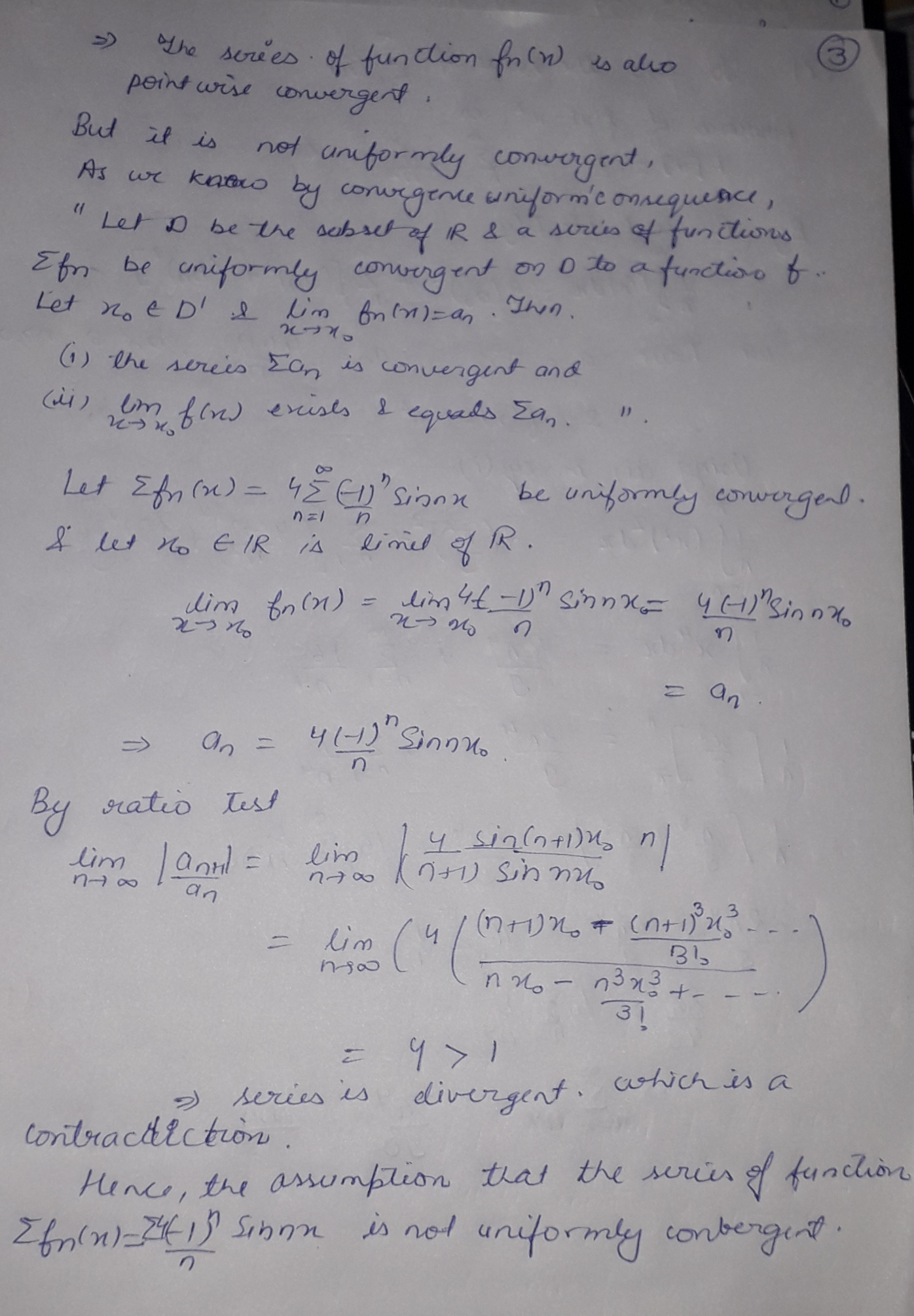##### Add Answer of: 6. Let f be a 20-periodic function, such that f(x) = x2 on (-1, ). The...
Similar Homework Help Questions
• ### (b) Determine for which values of x the following series converges and find the limit for...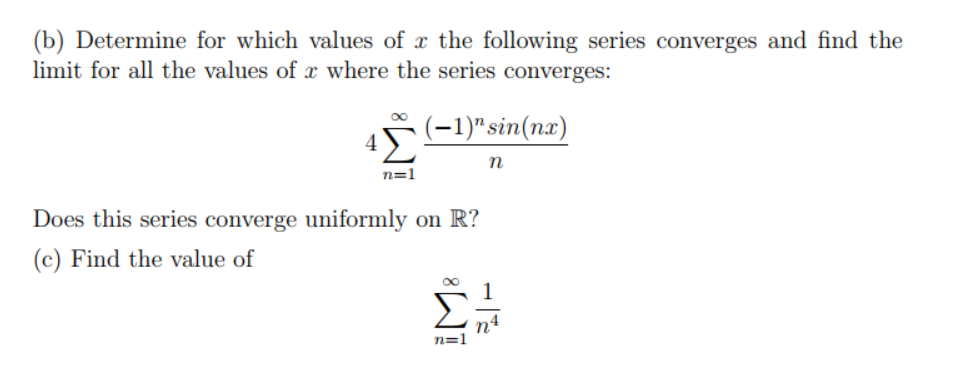(b) Determine for which values of x the following series converges and find the limit for all the values of x where the series converges: (-1)" sin(ne) n n=1 Does this series converge uniformly on R? (c) Find the value of M8 1 n4 n=1

• ### Partial Differential Equations: 2. (20 points total) (10 points) a) The functions fn(r)= + do they...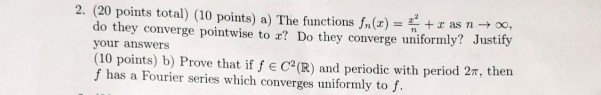Partial Differential Equations: 2. (20 points total) (10 points) a) The functions fn(r)= + do they converge pointwise to r? Do they converge uniformly? Justify r as n 0o. your answers (10 points) b) Prove that if f e C2(R) and periodic with period 2T, then fhas a Fourier series which converges uniformly to f. 2. (20 points total) (10 points) a) The functions fn(r)= + do they converge pointwise to r? Do they converge uniformly? Justify r as n...

• ### 4. For each n EN let fn: [0,1]R be given by if xE(0, otherwise fn(x) = (a) Find the function f : [0, 1] R to which {fn}...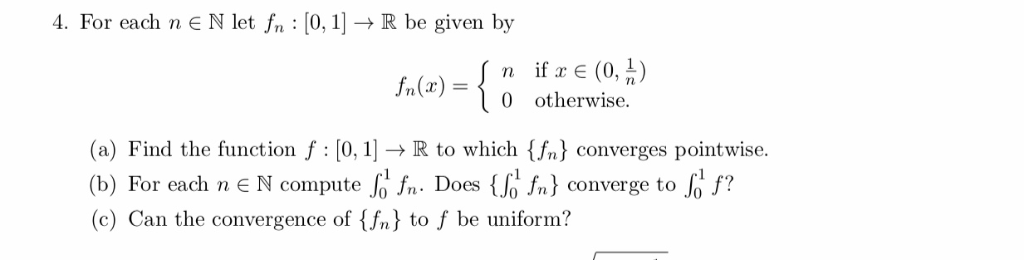4. For each n EN let fn: [0,1]R be given by if xE(0, otherwise fn(x) = (a) Find the function f : [0, 1] R to which {fn} converges pointwise. fn. Does {6 fn} converge to (b) For each n EN compute (c) Can the convergence of {fn} to f be uniform? 4. For each n EN let fn: [0,1]R be given by if xE(0, otherwise fn(x) = (a) Find the function f : [0, 1] R to which {fn}...

• ### Let f(x) be the 27-periodic function which is defined by f(x)-cos(x/4) for-π < x < 1. π. (a) Draw the graph of y f(x) over the interval-3π < x < 3π. Is f continuous on R? (b) Find the...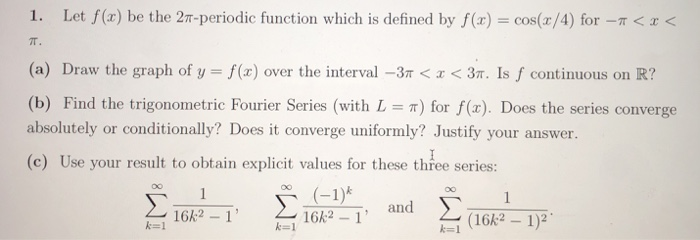Let f(x) be the 27-periodic function which is defined by f(x)-cos(x/4) for-π < x < 1. π. (a) Draw the graph of y f(x) over the interval-3π < x < 3π. Is f continuous on R? (b) Find the trigonometric Fourier Series (with L π) for f(x). Does the series converge absolutely or conditionally? Does it converge uniformly? Justify your answer. (c) Use your result to obtain explicit values for these three series: 16k2 1 16k2 1 (16k2 1)2 に1...

• ### Consider the function y = x2 for x E (-7,7) . a) Show that the Fourier series of this function is...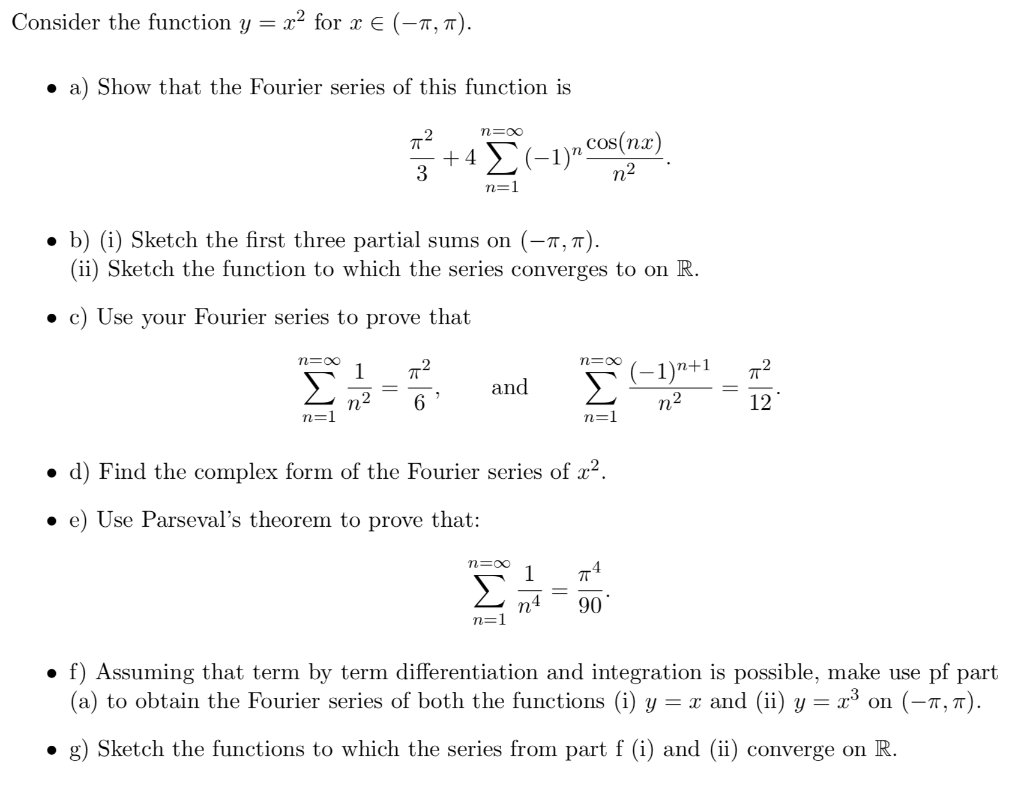Consider the function y = x2 for x E (-7,7) . a) Show that the Fourier series of this function is n cos(nz) . b) (i) Sketch the first three partial sums on (-π, π) (ii) Sketch the function to which the series converges to on R . c) Use your Fourier series to prove that 2and1)"+1T2 12 2 2 Tu . d) Find the complex form of the Fourier series of r2. . e) Use Parseval's theorem to prove...

• ### 3. For each n E N let fn : (1, 0) -+ R be given by f/(x) = Find the function f : (1, 0) - R to which {fn} converges poi...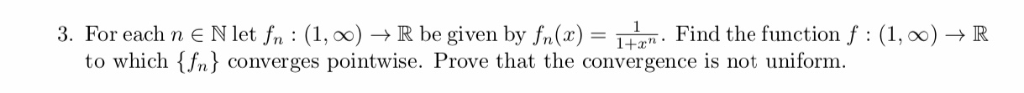3. For each n E N let fn : (1, 0) -+ R be given by f/(x) = Find the function f : (1, 0) - R to which {fn} converges pointwise. Prove that the convergence is not uniform 3. For each n E N let fn : (1, 0) -+ R be given by f/(x) = Find the function f : (1, 0) - R to which {fn} converges pointwise. Prove that the convergence is not uniform

• ### 1. Let f(x) be the 2T-periodic function which is defined by f(xcos(x/4) for -<< (a) Draw the graph of y = f(x) over the interval-3r < x < 3π. Is f continuous on R? (b) Find the trigonomet...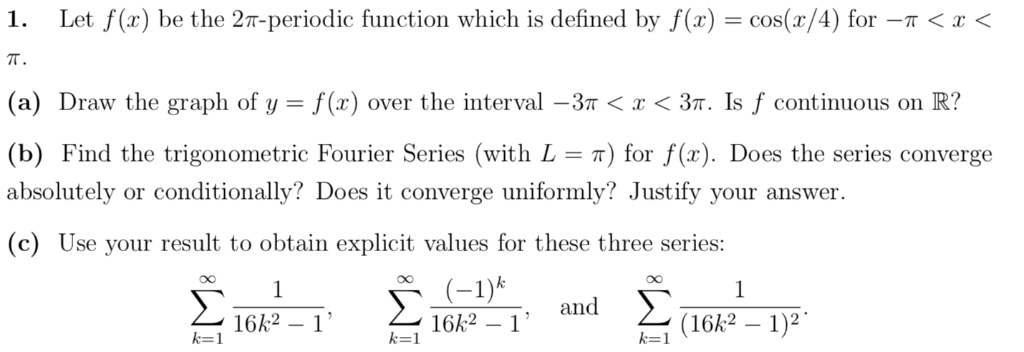1. Let f(x) be the 2T-periodic function which is defined by f(xcos(x/4) for -<< (a) Draw the graph of y = f(x) over the interval-3r < x < 3π. Is f continuous on R? (b) Find the trigonometric Fourier Series (with L = π) for f(x). Does the series converge absolutely or conditionally? Does it converge uniformly? Justify your answer. (c) Use your result to obtain explicit values for these three series: and , and 162 16k2-1" 16k2 1)2 に1...

• ### 1. (a) Let {fn}neN : [0,00) + R be a sequence of function define by: sin(nx)...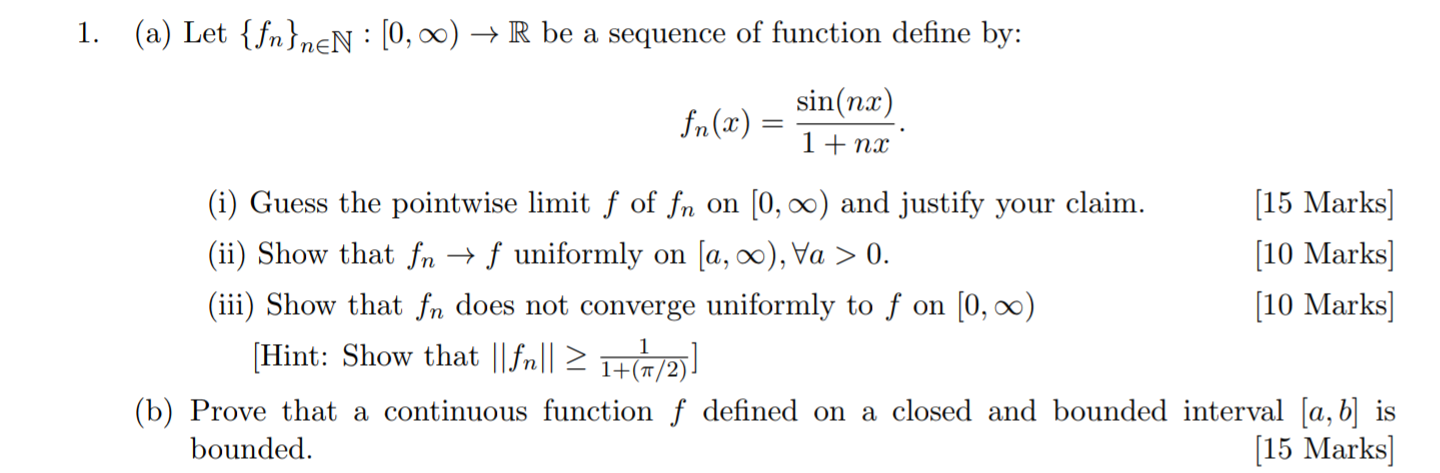1. (a) Let {fn}neN : [0,00) + R be a sequence of function define by: sin(nx) fn(x) 1+ nx (i) Guess the pointwise limit f of fn on (0,00) and justify your claim. [15 Marks] (ii) Show that fn + f uniformly on ſa, 00), Va > 0. [10 Marks) (iii) Show that fn does not converge uniformly to f on (0,00) [10 Marks] (Hint: Show that ||fr|| 21+(1/2) (b) Prove that a continuous function f defined on a closed...

• ### Find the pointwise limit function of the sequence fn(x)a) Find the pointwise limit function of the sequence fn(x)=*sin(x/n), 0<x<∞b) Determine the type of convergence of sequennce on 0<x<∞?(Dose the sequence converge uniformly or not?)

• ### Consider the series following series of functions ' sin(nx) 3 n-1 a) Show that the series is absolutely and uniformly convergent on the real axis. Let f be its summation function n sin(nx) b) Sho...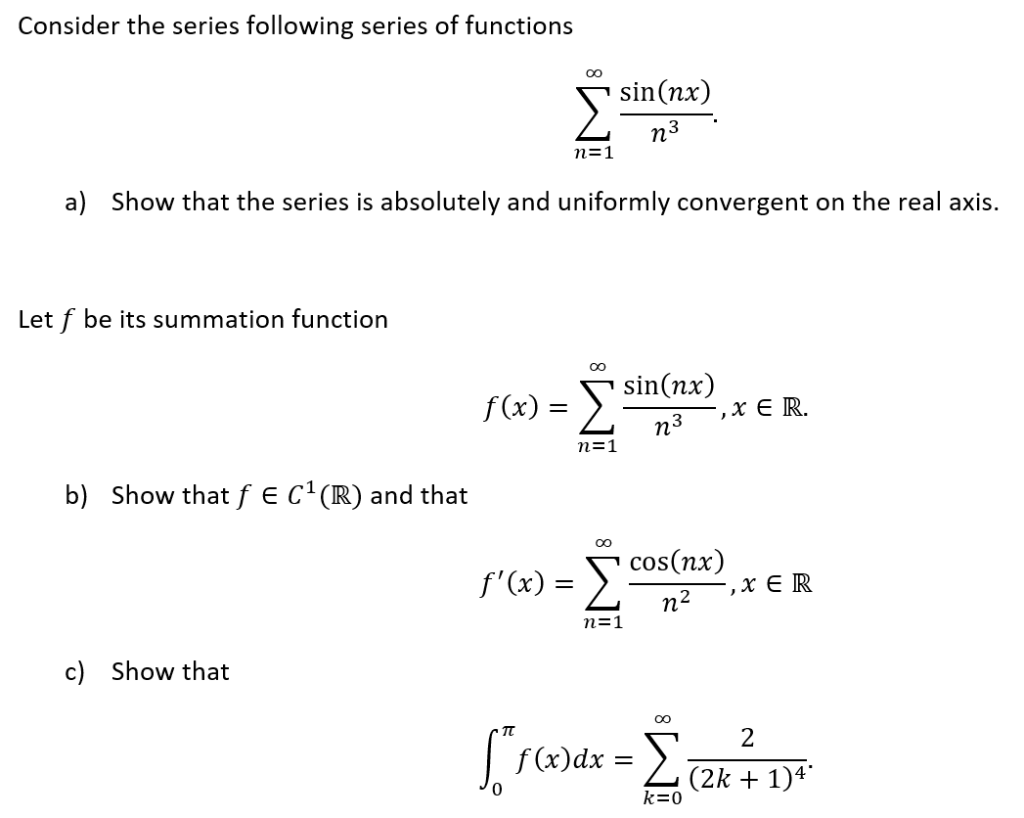Consider the series following series of functions ' sin(nx) 3 n-1 a) Show that the series is absolutely and uniformly convergent on the real axis. Let f be its summation function n sin(nx) b) Show that f E C(R) and that 1 cos(nx) f'(x)= 2-1 c) Show that 「 f#072821) f(x)dx = k=0 Consider the series following series of functions ' sin(nx) 3 n-1 a) Show that the series is absolutely and uniformly convergent on the real axis. Let f...

Free Homework App